Courses

# TEST: Addition (Give And Take) - 3

## 20 Questions MCQ Test Mathematics (Maths) for Class 3 | TEST: Addition (Give And Take) - 3

Description
This mock test of TEST: Addition (Give And Take) - 3 for Class 3 helps you for every Class 3 entrance exam. This contains 20 Multiple Choice Questions for Class 3 TEST: Addition (Give And Take) - 3 (mcq) to study with solutions a complete question bank. The solved questions answers in this TEST: Addition (Give And Take) - 3 quiz give you a good mix of easy questions and tough questions. Class 3 students definitely take this TEST: Addition (Give And Take) - 3 exercise for a better result in the exam. You can find other TEST: Addition (Give And Take) - 3 extra questions, long questions & short questions for Class 3 on EduRev as well by searching above.
QUESTION: 1

### Fill in the table below. The rule is to Add 18 to each term.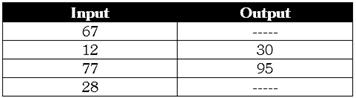Solution:

67 + 18 = 85 28 + 18 = 46

QUESTION: 2

### State whether the following statements are true or false. A. 5746 + 2222 is as same as 3984 + 3984 B. If we add 777 to 7777 we will get 8554 C. If we add 89 to 6789 we will get 6879 D. 3000 + 175 is 3 more than 2981 + 179

Solution:

TTFF 5746+2222=7968=3984+3984 7777+777=8554 6789 + 89 = 6878 3000 + 175 = 3175 while 2981 + 179 =3160

QUESTION: 3

### State whether the following statements are true or false 6896 + 3583 = 10478 If we add 333 + 5444 we will get 5777 if we add 5432 and 1234 we will get 5555 2222+1357=3579

Solution:

6896 + 3583 = 10479 333+5444=5777 5432+1234=6666 2222+1357=3579

QUESTION: 4

Surabhi has 14 m of ribbon with her. Sana has 15 m of ribbon. If they tie their ribbon together, what will be length of the new ribbon?

Solution:

Length of the new ribbon = 15 + 15 = 29

QUESTION: 5

Ajay is 18 years old. His brother Sumit is 5 years elder to him. How old is Sumit?

Solution:

18 + 5 = 23 years

QUESTION: 6

The table below shows the marks for top 4 students in class III for Mathematics and English".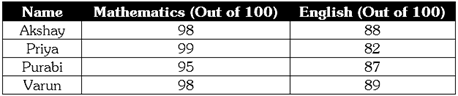Who has scored the highest total number of marks in both subjects?

Solution:

98 + 88 = 186 99 + 82 = 181 95 + 87 = 182 98+89=187

QUESTION: 7

The table below shows the number of stickers collected by 4 friends.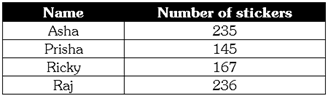How many stickers do Asha and Ricky have in all?

Solution:

235 + 167 = 402

QUESTION: 8

Hira has 40 pencils in a box. Sameer has 78 pencils in another box. How man pencils do Hira and Sameer have in all?

Solution:

40 + 78 = 118

QUESTION: 9

Ashima participated in a charity fair for a week. She had a stall of toys in th fair. Ashima sold 123 toy cars. 89 dolls and 100 balls in a week. How many toy did Ashima sell in all?

Solution:

123 + 89 + 100 = 312

QUESTION: 10

Hitesh drove 145 km on Monday and 340 km on Tuesday. How many kilometers did he drive in all?

Solution:

145 + 340 = 485

QUESTION: 11

Suveer works as mechanic at a service station. He repaired 150 cars and 167 motorcycles in 2 months. How many vehicles did Suveer repair in all?

Solution:

150 + 167 = 317

QUESTION: 12

Look at the route map below. A: Isha's house; B: Shop; C: Bus stop] Isha takes a bus from the bus stop. The bus is travelling towards Isha's house. The distance between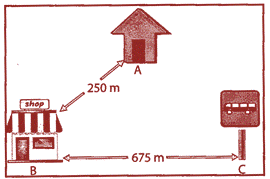Isha's house and shop is 250 m. The distance between Bus stand and shop is 675 m. The bus drops Isha at the shop and then she walks to her home. How much total distance did Isha cover?

Solution:

675+250= 925m

QUESTION: 13

The table below shows what 125 children eat for breakfast: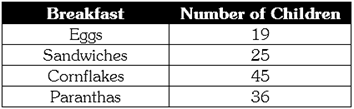How many children eat Cornflakes and Sandwiches?

Solution:

25+45=70

QUESTION: 14

Rakhi is 45 years old. Shiny is 35 years old. If Amey's age is equal to the sum of Rakhi's and Shiny's age, how old is Amey?

Solution:

45 + 35 = 80 years

QUESTION: 15

Look at the number grid below.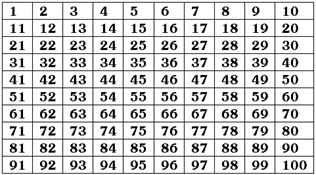Prachi starts from number 23. She takes 15 steps forward. On which number will she reach?

Solution:

23 + 15 = 38

QUESTION: 16

Look at the number grid given in above question.Ajay starts from number 45. How many steps will he have to take to reach number 50?

Solution:

45+5 =50

QUESTION: 17

Class 3 students of a school go for an excursion trip. The teacher takes 12 girl and 25 boys to a museum. How many tickets will they need to buy?

Solution:

Number of tickets =12 + 25 + 1 = 38 1 ticket needs to be bought for the class teacher.

QUESTION: 18

Rio, Seema and Sonia bought some books. Sonia bought 10 books. Ria bought 5 more than Sonia. Seema bought 3 more books than Ria. How many books did they buy in all?

Solution:

Ria bought 10 + 5 books =5
Seema bought 15 + 3 = 18 books 10 + 15 + 18 = 43 books.

QUESTION: 19

Find the missing digit. 5?-21=35

Solution:

Consider the unit digit in both numbers on LHS so '?' - 1 = 5 = >? = 6 56-21=35

QUESTION: 20

Find the missing digit. 906 - 5? 5 = 351

Solution:

906 - 555 = 351